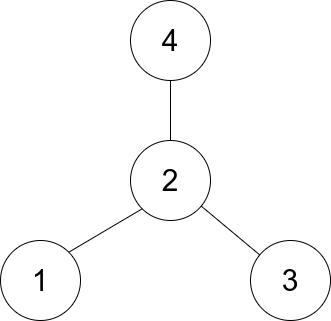# Find Center of Star Graph

## 1: U-nderstand

Understand what the interviewer is asking for by using test cases and questions about the problem.

• Established a set (2-3) of test cases to verify their own solution later.
• Established a set (1-2) of edge cases to verify their solution handles complexities.
• Have fully understood the problem and have no clarifying questions.
• Have you verified any Time/Space Constraints for this problem?
• Does the graph have to a connected graph?
• Yes, this graph need to be a connected graph. There would be no star graph if graph is disconnected.
• How many nodes could there be?
• Between 3 and 10000 nodes
• Are all node and edge pairs unique, there are no duplicate pairs correct?
• Yes all pairs are unique and there are no duplicate pairs
• What is the time and space constraints for this problem?
• Let's go with `O(1)` time and `O(1)` space.
``````HAPPY CASE
Input: edges = [[1,2],[2,3],[4,2]]
Output: 2
Explanation: As shown in the figure above, node 2 is connected to every other node, so 2 is the center.````````````Input: edges = [[1,2],[5,1],[1,3],[1,4]]
Output: 1

EDGE CASE
Input: edges = [[1,2],[2,3]]
Output: 2``````

## 2: M-atch

Match what this problem looks like to known categories of problems, e.g. Linked List or Dynamic Programming, and strategies or patterns in those categories.

For Graph Problems, common solution patterns include:

• DFS/BFS: This may is a connected graph, a DFS/BFS approach help us identify a node with n-1 connections.
• Adjacency Matrix: We can use an adjacency matrix to store the graph, but this will make the problem more complicated
• Topological Sort: This sort will make this problem more complicated.
• Union Find: Are there find and union operations here? Can you perform a find operation where you can determine which subset a particular element is in? This can be used for determining if two elements are in the same subset. Can you perform a union operation where you join two subsets into a single subset? Can you check if the two subsets belong to same set? If no, then we cannot perform union.

## 3: P-lan

Plan the solution with appropriate visualizations and pseudocode.

Approach #1:

General Idea: A valid start graph suggest that the center is connected to every edge, therefore we can get the center by seeing the comment element in the first 2 pairs.

``Create 2 sets and return the common element between the two sets.``

Approach #2:

General Idea: A valid start graph suggest that the center is connected to every edge, therefore we can get the center by seeing the comment element in the first 2 pairs.

``If the first element in the edge pair is in the second pair, then this is the common element and therefore the center node. Else it is the second element. ``

⚠️ Common Mistakes

• Generating a union set here, would work. However, there is more simple method.

## 4: I-mplement

Implement the code to solve the algorithm.

Approach #1:

``````class Solution:
def findCenter(self, edges: List[List[int]]) -> int:
# Create 2 sets and return the common element between the two sets.
return (set(edges) & set(edges)).pop()``````

Approach #2:

``````class Solution:
def findCenter(self, edges: List[List[int]]) -> int:
# If the first element in the edge pair is in the second pair, then this is the common element and therefore the center node. Else it is the second element.
return  edges if edges == edges or edges == edges else edges ``````
``````class Solution {
public int findCenter(int[][] e) {
// # If the first element in the edge pair is in the second pair, then this is the common element and therefore the center node. Else it is the second element.
return e == e || e == e ? e : e;
}
}``````

## 5: R-eview

Review the code by running specific example(s) and recording values (watchlist) of your code's variables along the way.

• Trace through your code with an input to check for the expected output
• Catch possible edge cases and off-by-one errors

## 6: E-valuate

Evaluate the performance of your algorithm and state any strong/weak or future potential work.

Assume `V` represents the number of vertices. Assume `E` represents the number of edges.

Approach #1 & #2 Have the same time and space complexity:

• Time Complexity: O(1) We only need to visit two edges.
• Space Complexity: O(1) We don't need to use additional memory.